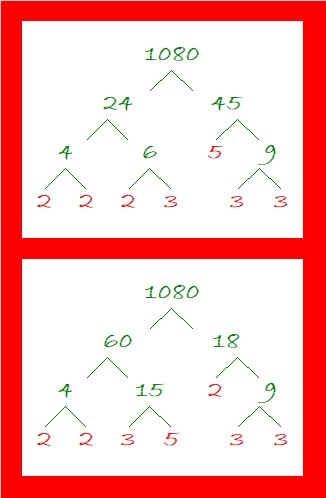# STOP! Look How Cool a Number 1080 Is!

Contents

### STOP Sign Geometry Fact:

What can I tell you about the number 1080?  Lots of things!

The sum of the interior angles of an eight-sided polygon such as a stop sign is 1080°.The sum of the interior angles of an octagon is 6(180°) = 1080°. Convex or Concave, it doesn’t matter, the sum of those interior angles of an eight-sided polygon will still be 1080°, as illustrated below:### Factor Trees for 1080:

Here are a couple of the MANY possible factor trees for 1080:### Factors of 1080:

There is only one number less than 1080 that has as many factors as 1080 does. What was that number? 840. How many factors does1080 have? 32. Wow!

• 1080 is a composite number.
• Prime factorization: 1080 = 2 × 2 × 2 × 3 × 3 × 3 × 5, which can be written 1080 = 2³ × 3³ × 5.
• The exponents in the prime factorization are 3, 3, and 1. Adding one to each and multiplying we get (3 + 1)(3 + 1)(1 + 1) ) = 4 × 4 × 2 = 32. Therefore 1080 has exactly 32 factors.
• Factors of 1080: 1, 2, 3, 4, 5, 6, 8, 9, 10, 12, 15, 18, 20, 24, 27, 30, 36, 40, 45, 54, 60, 72, 90, 108, 120, 135, 180, 216, 270, 360, 540, 1080
• Factor pairs: 1080 = 1 × 1080, 2 × 540, 3 × 360, 4 × 270, 5 × 216, 6 × 180, 8 × 135, 9 × 120, 10 × 108, 12 × 90, 15 × 72, 18 × 60, 20 × 54, 24 × 45, 27 × 40 or 30 × 36
• Taking the factor pair with the largest square number factor, we get √1080 = (√36)(√30) = 6√30 ≈ 32.863351080 has 28 composite factors and is the smallest number that can make that claim. (Of its 32 factors, all are composite numbers except 1, 2, 3, and 5). That’s more than 840’s 27 composite factors. (Its 32 factors minus 1, 2, 3, 5, and 7)

### Sum Difference Puzzles:

30 has four factor pairs. One of those pairs adds up to 13, and  another one subtracts to 13. Put the factors in the appropriate boxes in the first puzzle.

1080 has sixteen factor pairs. One of the factor pairs adds up to ­78, and a different one subtracts to 78. If you can identify those factor pairs, then you can solve the second puzzle!The second puzzle is really just the first puzzle in disguise. Why would I say that?

### Other Facts about the Number 1080:

1080 is the sum of these four consecutive prime numbers:
263 + 269 + 271 + 277 = 1080

1080 is the sum of four consecutive powers of three:
3⁶ + 3⁵ + 3⁴ + 3³ = 1080

1080 is the hypotenuse of a Pythagorean triple:
648-864-1080 which is (3-4-5) times 216

Note that 5(6³) = 5(216) = 1080 so 1080 is 500 in BASE 6.
It’s palindrome 252 in BASE 22 because 2(22²) + 5(22) + 2(1) = 1080,
UU in BASE 35 (U is 30 base 10) because 30(35) + 30(1) = 30(36) = 1080,
and it’s U0 in BASE 36 because 30(36) = 1080

And now I’ll STOP writing about how cool 1080 is.

## 6 thoughts on “STOP! Look How Cool a Number 1080 Is!”

1.adityaguharoy

I have heard of spoofs that show every number is cool (being the smallest uncool number is actually a cool property !), but I am glad to find some serious discussion on the numbers.

•ivasallay

Thank you! You’ve made my day!

•adityaguharoy

It’s pleasure to hear that you liked it. It is closely related to the Berry’s paradox.

2.adityaguharoy

By the way here is my website : https://scoutmathematics.wordpress.com/ where I plan to discuss some such things soon (by next week probably I will start such discussions).

•ivasallay

That sounds fun!

•adityaguharoy

Thank you, also see the new Problem of the Week news and send suggestions 🙂 if you want and also send problems.

This site uses Akismet to reduce spam. Learn how your comment data is processed.